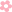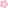APP下载# Vlookup函数最经典的12种用法 果断收藏！

Vlookup函数的教程网上已非常多了，而贴近工作用的Vlookup函数应用示例却很少。今天给大家带来一期Vlookup函数示例大全，希望能给大家的工作带来帮助。

【例1】查找个人信息

=VLOOKUP(\$B18,\$C\$7:\$H\$15,COLUMN(B1),0)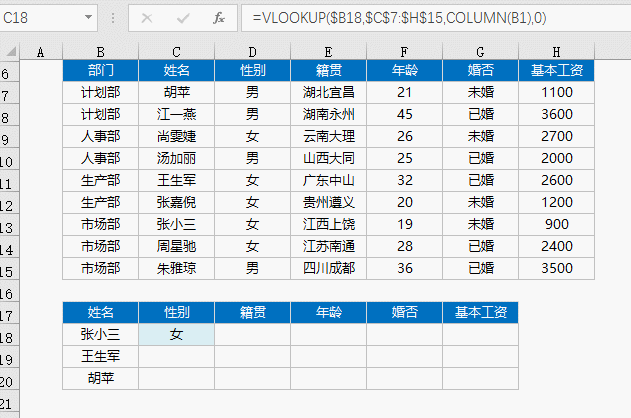【例2】合并两个表格

F2单元格公式

=VLOOKUP(B2,\$B\$14:\$F\$22,MATCH(F\$1,\$B\$13:\$F\$13,0))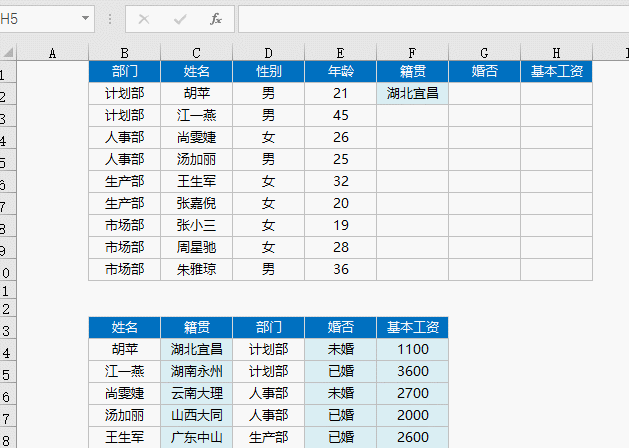【例3】让两个表格同类商品排序完全一样

F2单元格公式

=IFERROR(VLOOKUP(\$B2,\$B\$12:\$D\$16,COLUMN(A1),),"")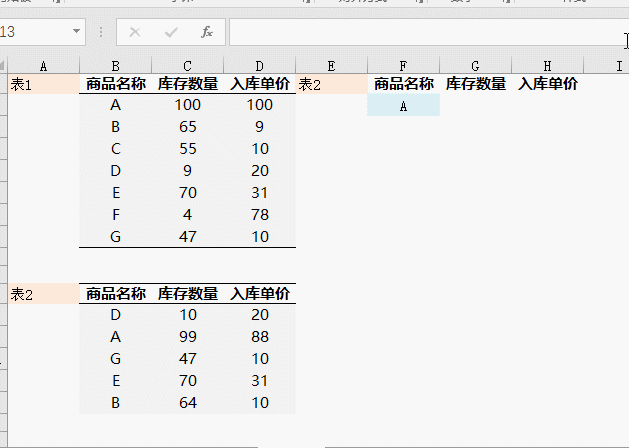【例4】制作工资条

=VLOOKUP(\$G96,\$A\$96:\$E\$104,COLUMN(B1),)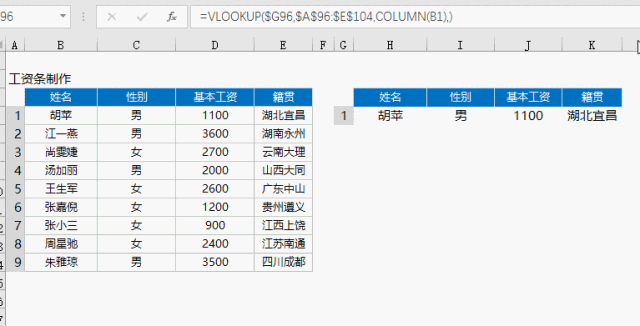【例5】计算个人所得税

=(G28-3500)*VLOOKUP(G28-3500,C28:E34,2)-VLOOKUP(G28-3500,C28:E34,3)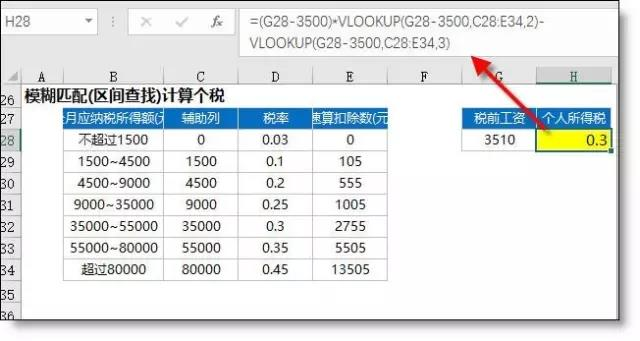【例6】模糊查找产品名称（包含查找）

=VLOOKUP("*"&F41&"*",\$B\$41:\$D\$47,3,0)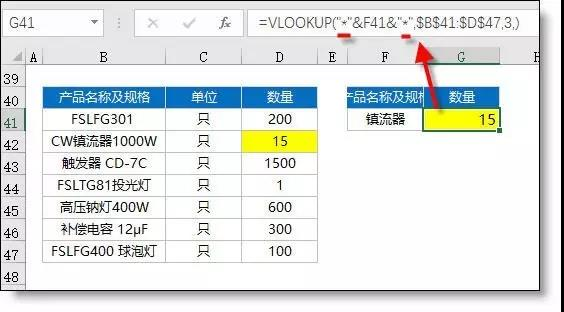【例7】带特殊符合的字符查找

=VLOOKUP(SUBSTITUTE(F52,"~","~~"),B\$52:C\$56,2,0)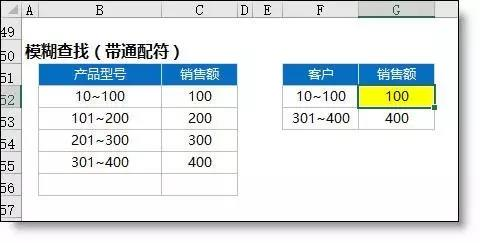【例8】逆向查找

=VLOOKUP(G61,IF({1,0},C61:C69,B61:B69),2,)

=INDEX(B60:B69,MATCH(G61,C60:C69,0))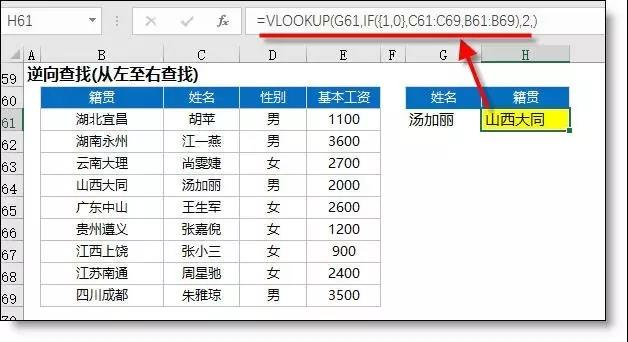【例9】16年与17年单价核对，表格核对（带通配符），结果显示多N 或 少N

=TEXT(VLOOKUP(SUBSTITUTE(B73,"*","~*"),\$F\$73:\$G\$92,2,)-C73,"少0.00;多0.00;")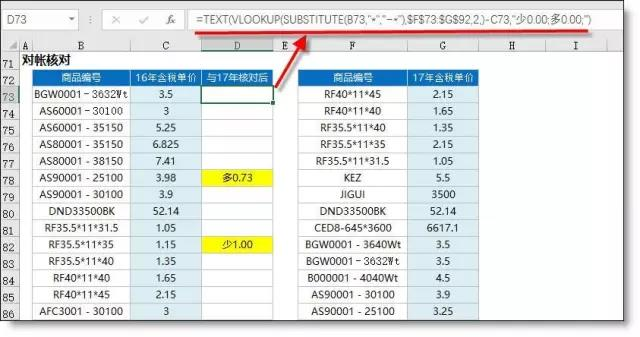【例10】Vlookup多条件查找

A112 =B112&C112

H112 =VLOOKUP(F112&G112,A112:D120,4,0)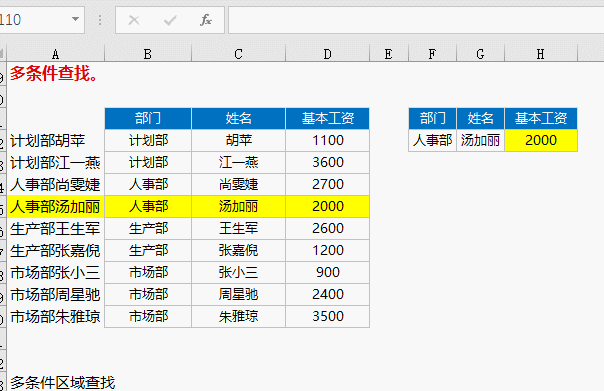【例11】库存单价的监控。销售单价>库存单价时填充红色背景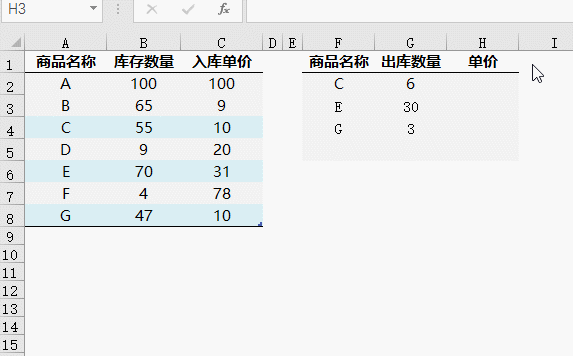=H2>VLOOKUP(F2,A:C,3,0)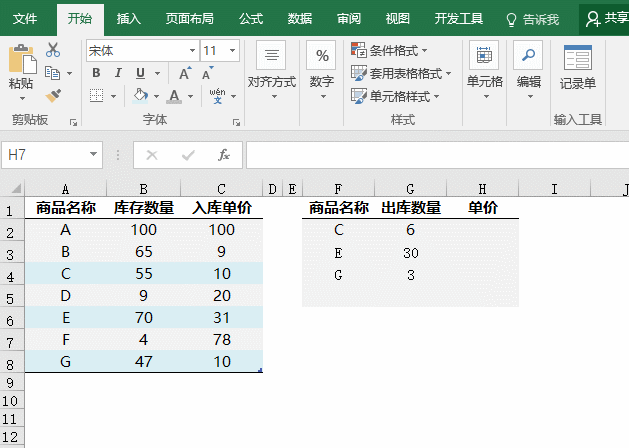【例12】银行存款最近30天到期提醒。表中要有某天汇总金额，也要有明细金额。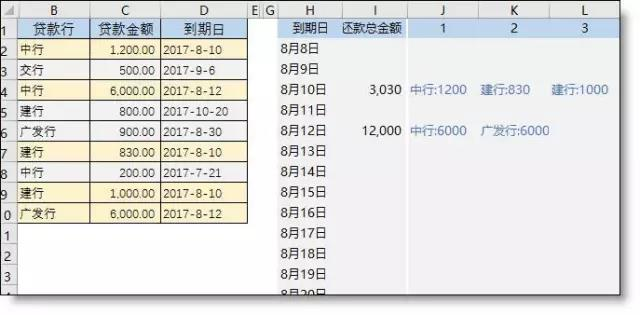=D2&"-"&COUNTIF(D\$2:D2,D2)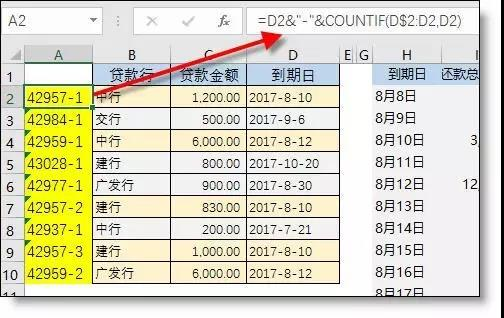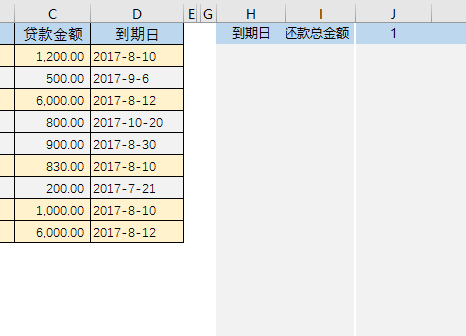=SUMIF(D:D,H2,C:C)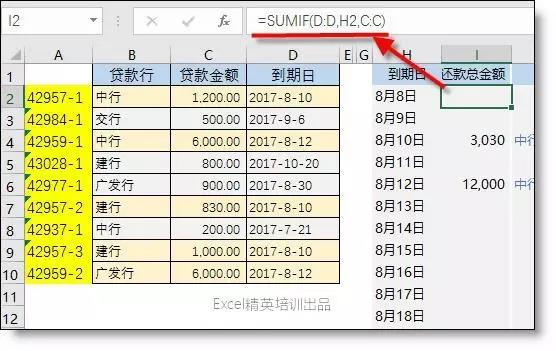J2单元格公式

=IFERROR(VLOOKUP(\$H2&"-"&J\$1,\$A:\$D,2,0)&":"&VLOOKUP(\$H2&"-"&J\$1,\$A:\$D,3,0),"")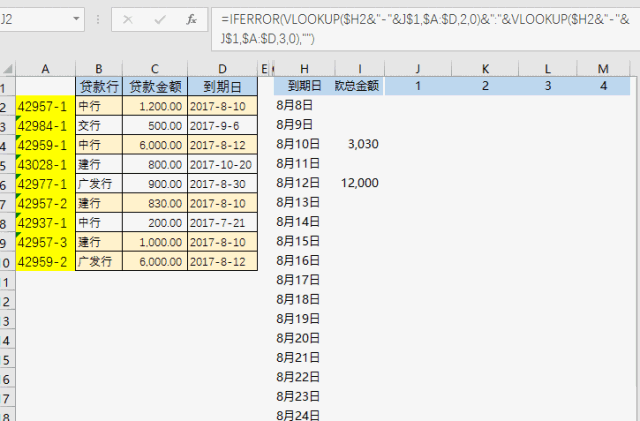## 实务学习指南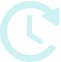距4月报税结束还有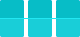天 能力测评 模拟实训 专家问答 资讯文章 财经法规 实务课程

## 学习方案

•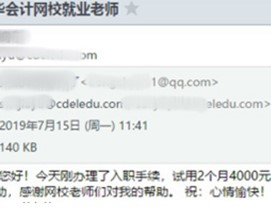37岁零基础转行做会计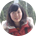会计新锐学员
•四十岁进入国企当财管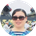财务主管训练营学员
•注会没考过也能进入事务所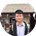事务所训练营学员
•考过中级的无经验小白成功就业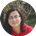会计新锐学员

## 限时免费资料

•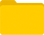财务表格

•纳税申报

•成本管理

•常用Excel

•实务资料库

## 加入会计精英实操群APP下载